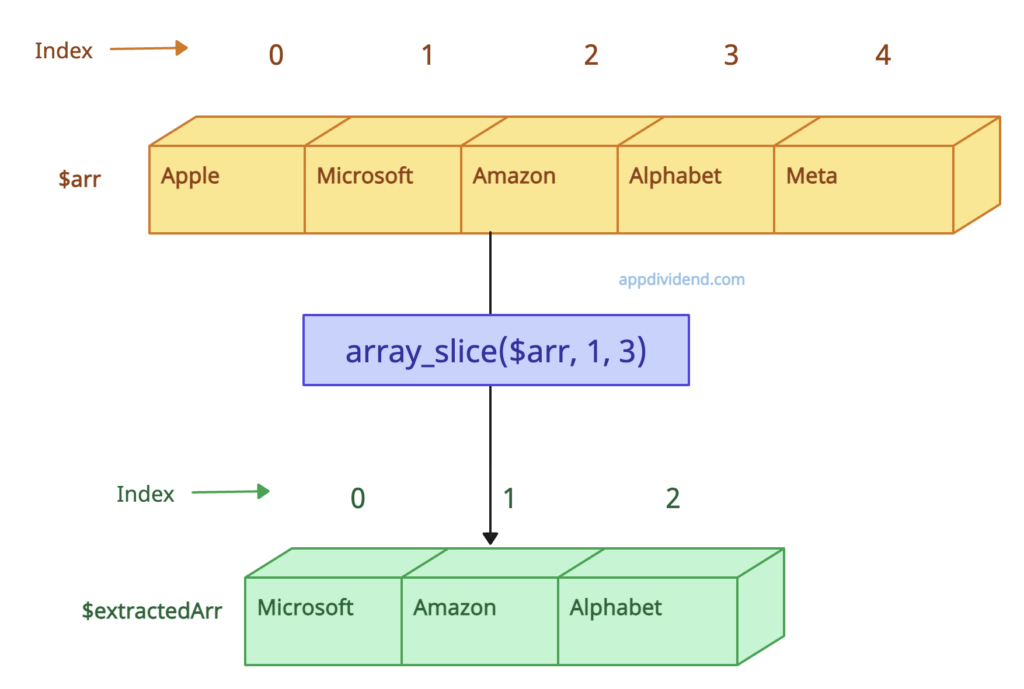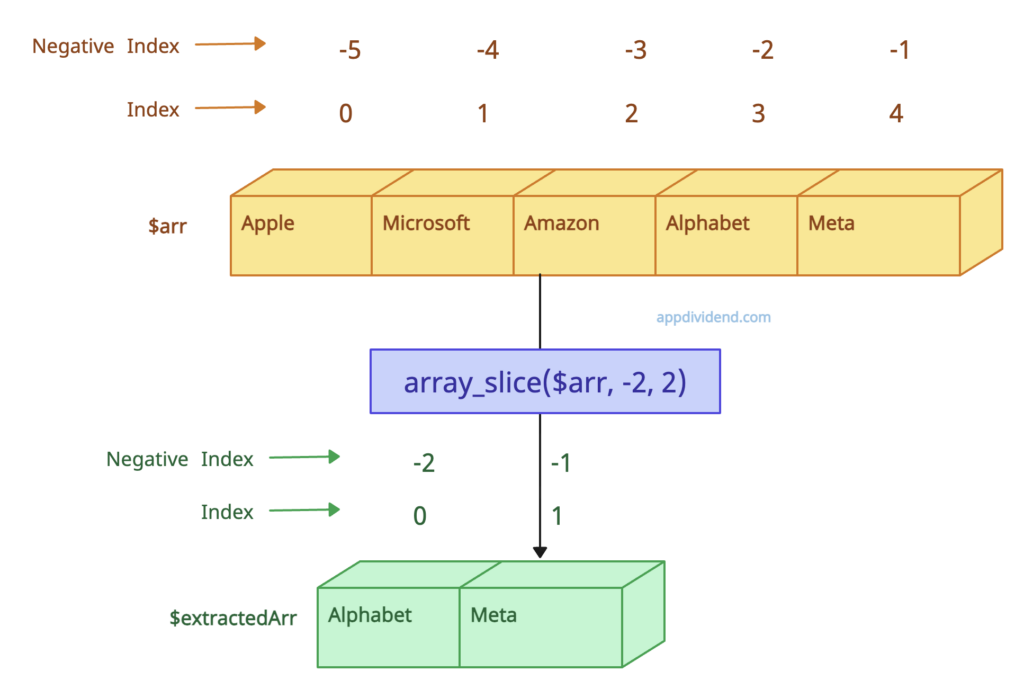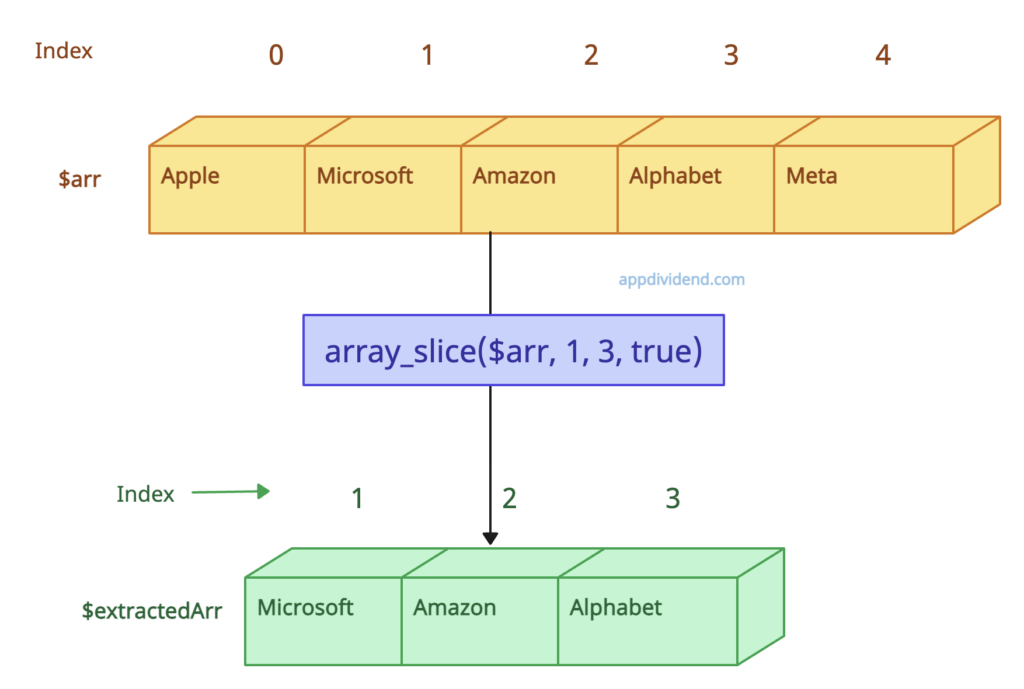# PHP array_slice() Function

PHP array_slice() function is used to extract a portion of an array.

## Syntax

``````array_slice(array,start,length,preserve)
``````

## Parameters

array(required): This parameter is an input array.

start(required): It Specifies where the function will start the slice: 0th index = the first element.

length(optional): It is a numeric value. If this value is set to a negative number, the function will start slicing from the end of the array.

preserve(optional): It can take boolean parameter:

1. true –  Preserve keys.
2. false -Reset keys(Default).

## Return value

It returns the selected or the sliced parts of the array.

## Example 1: How to Use array_slice() function

``````<?php

\$arr = ["Apple","Microsoft","Amazon","Alphabet","Meta"];

echo "Before slicing : \n";

print_r(\$arr);

\$extractedArr = array_slice(\$arr, 2);

echo "After slicing : \n";

print_r(\$extractedArr);``````

Output

``````Before slicing :
Array
(
 => Apple
 => Microsoft
 => Amazon
 => Alphabet
 => Meta
)
After slicing :
Array
(
 => Amazon
 => Alphabet
 => Meta
)``````

## Example 2: Using a length parameter``````<?php

\$arr = ["Apple","Microsoft","Amazon","Alphabet","Meta"];

echo "Before slicing : \n";

print_r(\$arr);

\$extractedArr = array_slice(\$arr, 1, 3);

echo "After slicing : \n";

print_r(\$extractedArr);
``````

Output

``````Before slicing :
Array
(
 => Apple
 => Microsoft
 => Amazon
 => Alphabet
 => Meta
)
After slicing :
Array
(
 => Microsoft
 => Amazon
 => Alphabet
)``````

## Example 3: Using a negative start parameter``````<?php

\$arr = ["Apple","Microsoft","Amazon","Alphabet","Meta"];

echo "Before slicing : \n";

print_r(\$arr);

\$extractedArr = array_slice(\$arr, -2, 2);

echo "After slicing : \n";

print_r(\$extractedArr);
``````

Output

``````Before slicing :
Array
(
 => Apple
 => Microsoft
 => Amazon
 => Alphabet
 => Meta
)
After slicing :
Array
(
 => Alphabet
 => Meta
)``````

In this example, The function extracts a slice from the array starting from the second-to-last element (as specified by -2) and includes up to 2 elements. So it returns 2 elements “Alphabet” and “Meta”.

## Example 4: Set the preserve parameter to true``````<?php

\$arr = ["Apple","Microsoft","Amazon","Alphabet","Meta"];

echo "Before slicing : \n";

print_r(\$arr);

\$extractedArr = array_slice(\$arr, 1, 3, true);

echo "After slicing : \n";

print_r(\$extractedArr);
``````

Output

``````Before slicing :
Array
(
 => Apple
 => Microsoft
 => Amazon
 => Alphabet
 => Meta
)
After slicing :
Array
(
 => Microsoft
 => Amazon
 => Alphabet
)``````

In this example, preserve parameter is true means the original array keys are preserved. This output shows three elements starting from the element with key 1 (“Microsoft“), including their original keys ,, and .

## Example 5: Using Associative Array

``````<?php

\$arr = [
'a' => 'Apple',
'b' => 'Microsoft',
'c' => 'Amazon',
'd' => 'Alphabet',

\$extractedArr = array_slice(\$arr, 0, 2);

print_r(\$extractedArr);``````

Output

``````Array
(
[a] => Apple
[b] => Microsoft
)``````

Categories PHP

This site uses Akismet to reduce spam. Learn how your comment data is processed.